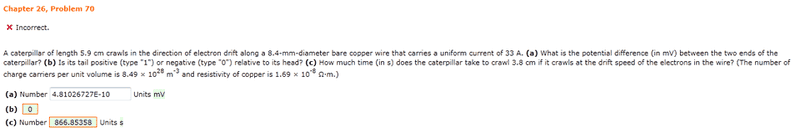# Ohm's Law Problem

## Homework StatementR = (Rou*A)/L
V = R/i

## The Attempt at a Solution

In this one I don't really understand how my answer to part a is wrong.
My Work: L = 5.9 cm = 5.9E-2 m
diameter = 8.4 mm = 8.4E-3 m ... radius = 4.2E-3 m ... A = pi*r^2 = pi(4.2E-3)^2 = 5.541769E-5 m^2
R = (Rou*A)/L = (1.69E-8 * 5.541769E-5)/(5.9E-2) = 1.5873882E-11 Ohms
V = R/i = (1.5873882E-11)/(33) = 4.81026727E-13 V = 4.81026727E-10 mV